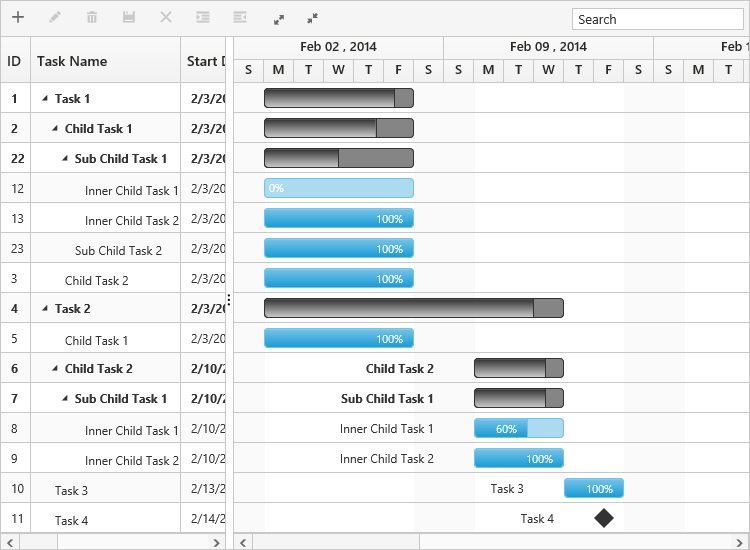# Data Binding

1 Mar 201711 minutes to read

Data binding is the process that establishes a connection between the application and different kinds of data sources such as business objects. And it is possible to bind local data and remote data in Gantt.

## Local Data Binding

In Local Data Binding, datasource for rendering the Gantt control is retrieved from the same application locally.

Two types of Data Binding are possible with Gantt control,

• Hierarchical datasource binding
• Self-referential data binding (Flat data)

### Hierarchical data-source binding

The following code example explains how to bind the hierarchical data in Gantt.

• CSHTML
• ``````<ej-gantt id="ganttSample" datasource="ViewBag.datasource"
start-date-mapping="StartDate"
end-date-mapping="EndDate"
duration-mapping="Duration"
progress-mapping="PercentDone">
</ejGantt>``````
• C#
• ``````List<DefaultData> list = new List<DefaultData>();
{
Id = 1,
Name = "Design",
StartDate = "02/10/2014",
EndDate = "02/14/2014",
Duration = 5,
PercentDone = 60,
Children = (new List<DefaultData>()
{
new DefaultData()
{
Id = 2,
Name = "Software Specification",
StartDate = "02/10/2014",
EndDate = "02/12/2014",
Duration = 4,
PercentDone = 60
},
new DefaultData()
{
Id = 3,
Name = "Develop prototype",
StartDate = "02/10/2014",
EndDate: "02/12/2014",
Duration = 4,
PercentDone = 70,
},
/...``````

The output of the above steps is as follows.### Self-referential data binding (Flat data)

Gantt can be rendered from self-referential data structures, by mapping the task ID and parent task ID fields.

• Task ID field- This field must contain unique values to identify the nodes. It should be mapped to the `task-id-mapping` property.
• Parent task ID field- This field must contain values to identify the parent nodes. It should be mapped to the `parent-task-id-mapping` property.
• C#
• ``````public ActionResult GanttSelfReference()
{
var DataSource = GetData();
ViewBag.datasource = DataSource;
return View();
}
public class SelfData
{
public string StartDate { get; set; }
public int Id { get; set; }
public string Name { get; set; }
public int Duration { get; set; }
public int PercentDone { get; set; }
public string Predescessor { get; set; }
public int? ParentId { get; set; }
}
public List<SelfData> GetData() {
List<SelfData> data = new List<SelfData>();
data.Add(new SelfData() { Id = 1, TaskName = "Task 1", StartDate = "02/03/2014", EndDate = "03/07/2014", Duration = 5 });
data.Add(new SelfData() { Id = 2, ParentId = 1, TaskName = "Child Task 1", StartDate = "02/03/2014", EndDate = "02/07/2014", Duration = 5 });
data.Add(new SelfData() { Id = 3, ParentId = 1, TaskName = "Child Task 2", StartDate = "02/03/2014", EndDate = "02/07/2014", Duration = 5, PercentDone = "100" });
data.Add(new SelfData() { Id = 22, ParentId = 2, TaskName = "Sub Child Task 1", StartDate = "02/03/2014", EndDate = "02/07/2014", Duration = 5 });
data.Add(new SelfData() { Id = 23, ParentId = 2, TaskName = "Sub Child Task 2", StartDate = "02/03/2014", EndDate = "02/07/2014", Duration = 5, PercentDone = "100" });
data.Add(new SelfData() { Id = 12, ParentId = 22, TaskName = "Inner Child Task 1", StartDate = "02/03/2014", EndDate = "02/07/2014", Duration = 5 });
data.Add(new SelfData() { Id = 13, ParentId = 22, TaskName = "Inner Child Task 2", StartDate = "02/03/2014", EndDate = "02/07/2014", Duration = 5, PercentDone = "100" });
data.Add(new SelfData() { Id = 4, TaskName = "Task 2", StartDate = "02/03/2014", EndDate = "02/07/2014", Duration = 5, PercentDone = "100" });
data.Add(new SelfData() { Id = 5, ParentId = 4, TaskName = "Child Task 1", StartDate = "02/03/2014", EndDate = "02/07/2014", Duration = 5, PercentDone = "100" });
data.Add(new SelfData() { Id = 6, ParentId = 4, TaskName = "Child Task 2", StartDate = "02/07/2014", EndDate = "02/07/2014", Duration = 5 });
data.Add(new SelfData() { Id = 7, ParentId = 6, TaskName = "Sub Child Task 1", StartDate = "02/07/2014", EndDate = "02/07/2014", Duration = 5 });
data.Add(new SelfData() { Id = 8, ParentId = 7, TaskName = "Inner Child Task 1", StartDate = "02/10/2014", EndDate = "02/12/2014", Duration = 3, PercentDone = "60" });
data.Add(new SelfData() { Id = 9, ParentId = 7, TaskName = "Inner Child Task 2", StartDate = "02/10/2014", EndDate = "02/12/2014", Duration = 3, PercentDone = "100" });
data.Add(new SelfData() { Id = 10, TaskName = "Task 3", StartDate = "02/13/2014", EndDate = "02/14/2014", Duration = 2, PercentDone = "100" });
data.Add(new SelfData() { Id = 11, TaskName = "Task 4", StartDate = "02/14/2014", EndDate = "02/14/2014", Duration = 0 });
return data;
}``````
• CSHTML
• ``````<ej-gantt id="ganttSample" datasource="ViewBag.datasource"